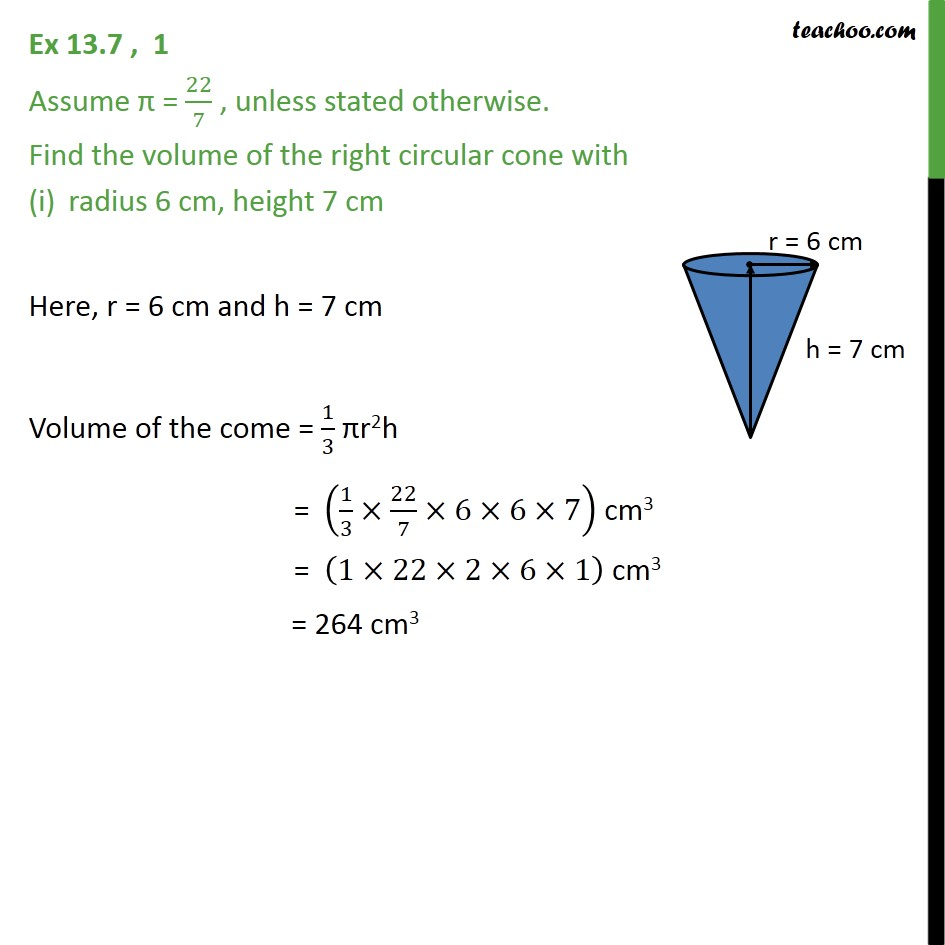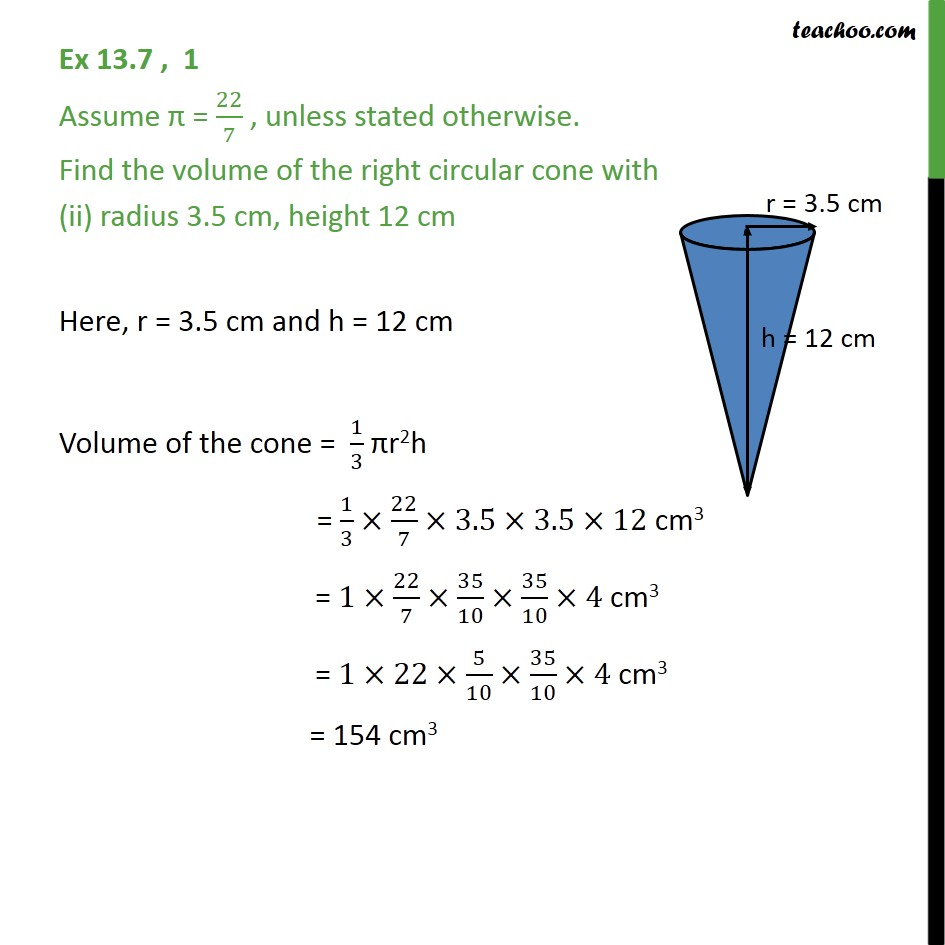Subscribe to our Youtube Channel - https://you.tube/teachoo

1. Chapter 13 Class 9 Surface Areas and Volumes
2. Serial order wise
3. Ex 13.7

Transcript

Ex 13.7 , 1 Assume = 22/7 , unless stated otherwise. Find the volume of the right circular cone with radius 6 cm, height 7 cm Here, r = 6 cm and h = 7 cm Volume of the come = 1/3 r2h = (1/3 22/7 6 6 7) cm3 = (1 22 2 6 1) cm3 = 264 cm3 Ex 13.7 , 1 Assume = 22/7 , unless stated otherwise. Find the volume of the right circular cone with (ii) radius 3.5 cm, height 12 cm Here, r = 3.5 cm and h = 12 cm Volume of the cone = 1/3 r2h = 1/3 22/7 3.5 3.5 12 cm3 = 1 22/7 35/10 35/10 4 cm3 = 1 22 5/10 35/10 4 cm3 = 154 cm3

Ex 13.7